# Samacheer Kalvi 9th Maths Book Solutions Chapter 2 | Real Numbers Answers For Tamil Nadu Board

Samacheer Kalvi 9th Maths Book Solutions Chapter 2 – Real Numbers is available here. The Samacheer Kalvi 9th Maths book answers of Chapter 2, available at BYJU’S, contain step by step explanations designed by our mathematics experts. All these important questions are based on the new pattern prescribed by the Tamil Nadu board. Students can also get the solutions of other chapters on samacheer kalvi 9th maths solutions. Students can first solve the Samacheer Kalvi 9th Maths Book Chapter 2 questions and then refer to the solutions to self-gauge one’s performance.

## Samacheer Kalvi Class 9 Maths Chapter 2 Solutions

Chapter 2 of the Samacheer Kalvi 9th Maths guide will help the students to solve problems related to the types of numbers, denseness property of rational numbers, irrational numbers, irrational numbers on the number line, decimal representation of a rational number, conversion of non-terminating and recurring decimals into rational numbers, decimal representation to identify irrational numbers, real numbers, the real number line, radical notation, fractional index, surds, the order of a surd, laws of radicals, basic operations on surds, rationalisation of surds, scientific notation, the arithmetic of numbers in scientific notation.

### Samacheer Kalvi 9th Maths Chapter 2: Real Numbers Book Exercise 2.1 Questions and Solutions

Question 1: Which arrow best shows the position of 11 / 3 on the number line?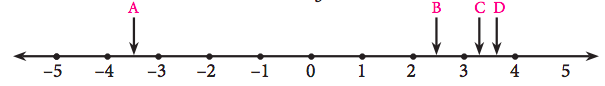Solution:

11 / 3 = 3.666….. = 3.7 (approximately)

∴ The arrow D best shows the position of 11 / 3 on the number line.

Question 2: Find any three rational numbers between [-7 / 11] and [2 / 11].

Solution:

The rational numbers between [-7 / 11] and [2 / 11] are -6 / 11, -5 / 11, -4 / 11, -3 / 11, -2 / 11, -1 / 11, 0 / 11, 1 / 11.

Question 3: Find any five rational numbers between

[i] 1 / 4 and 1 / 5

[ii] 0.1 and 0.11

[iii] -1 and -2

Solution:

[i] a = 1 / 4, b = 1 / 5

To find the 1st rational number between a and b, first, find the average of a and b.

c = (a + b) / 2

1st rational number

c = [(1 / 4) + (1 / 5)] / 2

= [( 5 + 4) / 20] / 2

= 9 / 40

2nd rational number

c = [(1 / 4) + (9 / 40)] / 2

= [(10 + 9) / 40] / 2

= 19 / 80

3rd rational number :

= [(1 / 4) + (19 / 80)] / 2

= (20 + 19) / 160

= 39 / 160

4th rational number :

= [(1 / 4) + (39 / 160)] / 2

= (40 + 39) / 320

= 79 / 320

5th rational number :

= [(1 / 4) + (79 / 320)] / 2

= (80 + 119) / 640

= 159 / 640

Hence the 5 rational numbers are 9 / 40, 19 / 80, 39 / 160, 79 / 320, 159 / 640.

[ii] 0.1 and 0.11

The rational numbers between 0.1 and 0.11 are 0.101, 0.102, 0.103, 0.104, 0.105, 0.106, ……….

[iii] -1 and -2

Let a, b, c, d, e be the rational numbers between -1 and -2.

a = [-1 + (-2)] / 2 = -3 / 2

b = [-1 + (-3 / 2)] / 2 = -5 / 4

c = [-1 + (-5 / 4)] / 2 = -9 / 8

d = [-1 + (-9 / 8)] / 2 = -17 / 16

e = [-1 + (-17 / 16)] / 2 = -33 / 32

Hence 5 rational numbers between -1 and -2 are -3 / 2, -5 / 4, -9 / 8, -17 / 16 and -33 / 32.

### Samacheer Kalvi 9th Maths Chapter 2: Real Numbers Book Exercise 2.2 Questions and Solutions

Question 1: Express the following rational numbers into decimal and state the kind of decimal expansion

(i) 2 / 7

(ii) -5 (3 / 11)

(iii) 22 / 3

(iv) 327 / 200

Solution:

(i) 2 / 7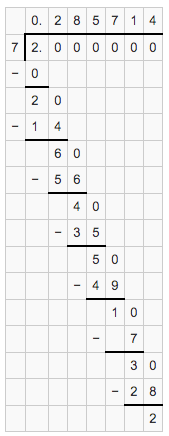2 / 7 = 0.28714………..

The given fraction has a non-terminating and recurring decimal expansion.

(ii) (-5 3 / 11) = (-58 / 11)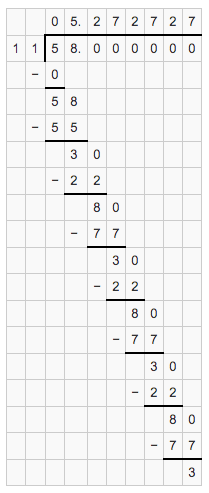-58 / 11 = 5.2727……….

The given fraction has a non-terminating and recurring decimal expansion.

(iii) 22 / 3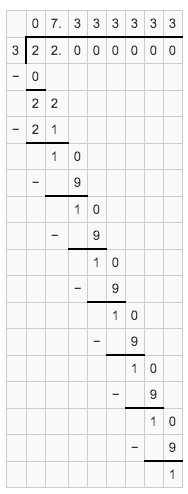22 / 3 = 7.3333……….

The given fraction has a non-terminating and recurring decimal expansion.

(iv) 327 / 200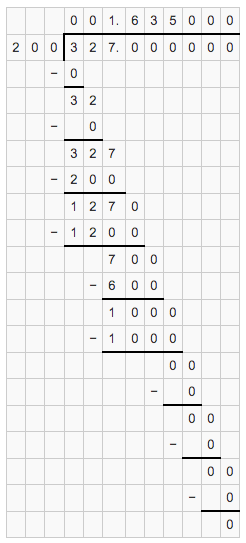327 / 200 = 1.635

The given fraction has a terminating decimal expansion.

Question 2: Express 100 / 13 in decimal form. Find the length of the period of decimals.

Solution: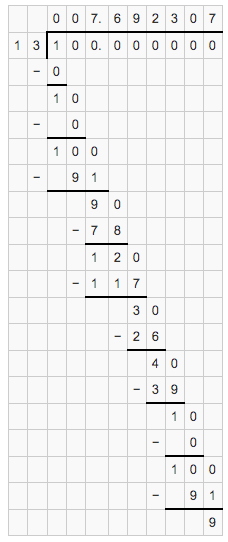100 / 13 has a length of period 6.

Question 3: Express the rational number 1 / 33 in recurring decimal form by using the recurring decimal expansion of 1 / 11. Hence write 71 / 33 in recurring decimal form.

Solution:

The recurring decimal expansion of 1 / 11 = 0.09090909….

1 / 33 = 0.03030303…..

71 / 33 = 2 + (5 / 33)

= 2 + (5 * [1 / 33])

= 2 + (5 * 0.030303…..)

= 2 + 0.151515

= 2.15151515 [recurring decimal]

Question 4: Express the following decimal expression into rational numbers.

[i]

$$\begin{array}{l}0.\overline{24}\end{array}$$

[ii]

$$\begin{array}{l}2.\overline{327}\end{array}$$

[iii] -5.132

[iv]

$$\begin{array}{l}3.1\overline{7}\end{array}$$

[v]

$$\begin{array}{l}17.\overline{215}\end{array}$$

[vi]

$$\begin{array}{l}-21.213\overline{7}\end{array}$$

Solution:

[i]
$$\begin{array}{l}0.\overline{24}\end{array}$$

Let x = 0.242424………… —–(1)

Since the bar is for two digits, multiply (1) by 100 on both sides.

100 x = 24.2424………..——(2)

Equation (2) – (1)

100x – x = 24.2424……- 0.2424………..

99x = 24

x = 24 / 99

x = 8 / 33

The rational form of the given decimal is 8 / 33.

[ii]
$$\begin{array}{l}2.\overline{327}\end{array}$$

Let x = 2.327327………… —–(1)

Since the bar is for three digits, multiply (1) by 1000 on both sides.

1000x = 2327.327327………..——(2)

Equation (2) – (1)

1000x – x = 2327.327327…..- 2.327327……..

999x = 2325

x = 2325 / 999

x = 775 / 333

The rational form of the given decimal is 775 / 333.

[iii] -5.132

The decimal -5.132 is a terminating one. There are three digits after the decimal. Multiply the numerator and denominator by 1000.

-5.132 = -5132 / 1000

= -2566 / 500

= -1283 / 250

[iv]
$$\begin{array}{l}3.1\overline{7}\end{array}$$

Let x = 3.177777……….. —–(1)

Since the bar is for one digit, multiply (1) by 10 on both sides.

10x = 31.77777………..——(2)

Equation (2) – (1)

10x – x = 31.7777…..- 3.17777……..

9x = 28.6

x = 28.6 / 9

Multiply both numerator and denominator by 10.

x = 286 / 90

x = 143 / 45

The fractional form of the given decimal is 143 / 45.

[v]
$$\begin{array}{l}17.2\overline{15}\end{array}$$

Let x = 17.2151515……….. —–(1)

Since the bar is for two digits, multiply (1) by 100 on both sides.

100x = 1721.51515………..——(2)

Equation (2) – (1)

100x – x = 1721.51515………. – 17.2151515………..

99x = 1704.3

x = 1704.3 / 99

Multiply both numerator and denominator by 10.

x = 17043 / 990

x = 5681 / 330

The fractional form of the given decimal is 5681 / 330.

[vi]
$$\begin{array}{l}-21.213\overline{7}\end{array}$$

Let x = 21.213777……….. —–(1)

Since the bar is for two digits, multiply (1) by 100 on both sides.

10x = 212.137777………..——(2)

Equation (2) – (1)

10x – x = 212.137777……….. – 21.213777………..

9x = 191.92

x = 191.924 / 9

x = 190924 / 9000

x = 47731 / 2250

Question 5: Without actual division, find which of the following rational numbers have terminating decimal expansion.

(i) 7 / 128

(ii) 21 / 15

(iii) 4 (9 / 35)

(iv) 219 / 2200

Solution:

(i) 7 / 128

128 = 27

7 / 128 = 7 / 27

The denominator is of the form 2m x 5n, hence, the given fraction is a terminating decimal.

(ii) 21/15

21 / 15 = 7 / 5

The denominator is not of the form 2m x 5n, hence, the given fraction is a non-terminating decimal.

(iii) 4 (9 / 35)

4 (9 / 35) = 149 / 35

35 = 7 x 5

The denominator is not in the form 2m x 5n, hence, the given fraction is a non terminating decimal.

(iv) 219 / 2200

2200 = 52 x 23 x 11

The denominator is in the form 2m x 5n, hence, the given fraction is non terminating decimal.

### Samacheer Kalvi 9th Maths Chapter 2: Real Numbers Book Exercise 2.3 Questions and Solutions

Question 1: Represent the following irrational numbers on the number line.

[i] √3

[ii] √4.7

[iii] √6.5

Solution:

[i] √3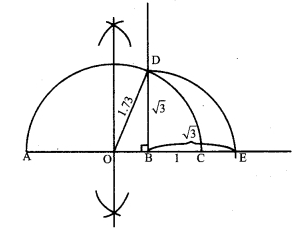(i) A line is drawn and point A is marked on it.

(ii) Point B is marked such that AB = 3cm.

(iii) Mark a point C on this line such that BC = 1 unit. Point Cis marked on the line such that BC = 1cm.

(iv) Let O be the perpendicular bisector of AC and find the midpoint of AC.

(v) A semicircle is drawn with O as its centre and OC = OA is the radius.

(vi) A line BD is drawn such that it is perpendicular to AB at B.

(vii) Here, BD = √3. It is marked on the number line as the value of BE is equal to BD = √3.

[ii] √4.7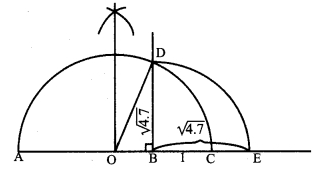(i) A line is drawn and point A is marked on it.

(ii) Point B is marked such that AB = 4.7 cm.

(iii) Point C is marked on the line such that BC = 1 unit.

(iv) Let O be the perpendicular bisector of AC and find the midpoint of AC.

(v) A semicircle is drawn with O as the centre such that OC = OA is the radius.

(vi) A line BD is drawn such that it is perpendicular to AB at B.

(vii) Here BD = √4.7. It is marked on the number line as the values of BE is equal to BD = √4.7

[iii] √6.5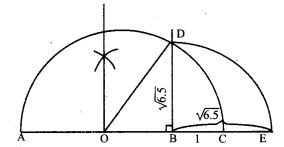(i) A line is drawn and point A is marked.

(ii) Point B is marked such that AB = 6.5 cm.

(iii) Point C is marked on the line such that BC = 1 unit.

(iv) Let O be the perpendicular bisector of AC and find the midpoint of AC.

(v) A semicircle is drawn with O as centre such that OC = OA is the radius.

(vi) A line BD is drawn such that it is perpendicular to AB at B.

(vii) Here BD = √6.5. It is marked on the number line as the value of BE is equal to BD = √6.5.

Question 2: Find any two irrational numbers between

(i) 0.3010011000111…. and 0.3020020002….

(ii) 6 / 7 and 12 / 13

(iii) √2 and √3

Solution:

(i) 0.3010011000111…. and 0.3020020002….

The two irrational numbers between 0.3010011000111…. and 0.3020020002…. are 0.301202200222 …., 0.301303300333…..

(ii) 6 / 7 and 12 / 13

6 / 7 = 0.857142…

12 / 13 = 0.923076

The two irrational numbers between 6 / 7 and 12 / 13 are 0.8616611666111…… and 0.8717711777111…..

(iii) √2 and √3

√2 = 1.414

√3 = 1.732

The two irrational numbers between √2 and √3 are 1.5155…. and 1.6166…….

Question 3: Find any two rational numbers between 2.2360679….. and 2.236505500….

Solution:

The two rational numbers between 2.2360679….. and 2.236505500…. are 2.2362 and 2.2363.